# A Level Mechanics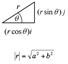Mechanics Essential Notes for the NEW A level Syllabus 2017 The Key Mechanics topics reduced down to a few sides. More than just the equations but good tips and techniques as written by some of our Yr12 students. PDF here. Versions of the old notes for AQA Mechanics 1 here (PDF here) and AQA Mechanics 2 here (PDF here).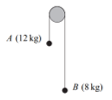Connected Particles Two masses hanging over a pulley, one mass hanging and another on a rough surface or a car and a trailer. These are all variations of the same mechanics, connected-particles, principle. This document demonstrates how. PDF here.How To Approach Mechanics Questions Students typically find it difficult to know where to begin with mechanics questions so here's a brief guide, some suggestions and common approaches and clues to completing such questions. PDF here.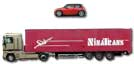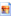Lorry Vs Mini (or as Ppt) Simple image file, consider attempting to push each vehicle and suddenly f=ma makes sense! The ppt has a vertical version of the image too.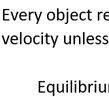Newtons Laws of Motion Notes on Newton's Laws of Motion PDF here.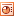Vector Forces in Equilibrium A series of questions of progressive difficulty, beginning with straightforward trig and building up to resolving and finding resultant forces from systems of three forces. PDF Here.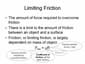Friction A Level Mechanics course notes on 'Friction'.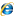Masses and Springs Lab A great external website demonstration of the conversions between GPE, KE and EPE and remaining constant as a whole.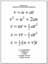Velocity & Acceleration Equations AS Level Mechanics 1, Velocity and Acceleration equations. Print them out on a big colourful sheet and learn them! PDF here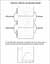Distance, Velocity, Acceleration Graphs Mechanics cribsheet to show how distance, velocity, accereation graphs are connected. PDF here.Vector Forces in Equilibrium Three sets of progressively more difficult questions on resolving vectors in equilibriumPDF here.